Latest Banking jobs   »

# Reasoning Ability Quiz For Bank Foundation 2023 – 28th March

Directions (1-3): Study the information carefully and answer the questions given below.
There are seven persons- P, Q, R, S, T, U and V in a family. There are two married couples and three generations in this family. Q is father-in-law of T. P is mother of R. S is nephew of T. R is niece of U. V is brother-in-law of T. U is mother of S.

Q1. Who among the following is father of R?
(a) P
(b) V
(c) T
(d) Q
(e) None of these

Q2. Who among the following are children of Q?
(a) T, U
(b) S, R
(c) V, P
(d) T, S
(e) None of these

Q3. How is U related to Q?
(a) Daughter-in-law
(b) Father-in-law
(c) Daughter
(d) Mother-in-law
(e) None of these

Directions (4-5): Study the following information and answer the questions given below.
A family consists of eight members A, B, C, D, E, F, G and H. B is the son of C but C is not the mother of B. A and C are married couple. E is the brother of C. D is the daughter of A and F is the brother of A. G is the father-in-law of A. B is grandson of H. G is a married man.

Q4. How is H related to A?
(a) father
(b) father-in-law
(c) Sister
(d) mother-in-law
(e) mother

Q5. How is D related to F?
(a) Son
(b)Uncle
(c) Daughter
(d) Niece
(e) Nephew

Direction (6-10): The following questions are based on the five three-digit numbers given below.

713 486 635 562 875

Q6. If the second and third digits of each number are added then the resulting sum of which number is divisible by 3?
(a) 486
(b) 713
(c) 562
(d) 875
(e) 635

Q7. If in each number second and third digits are interchanged then how many odd numbers will be formed?
(a) 2
(b) 3
(c) 5
(d) 1
(e) 4

Q8. If in each number all digits are arranged in ascending order from left to right, then which of the following will be the highest?
(a) 486
(b) 713
(c) 562
(d) 875
(e) 635

Q9. If in each number first and third digits are interchanged, then how many even numbers will be formed?
(a) 2
(b) 3
(c) 5
(d) 1
(e) 4

Q10. If in each number all digits are arranged in descending order from left to right, then which of the following number will be the second lowest?
(a) 486
(b) 713
(c) 562
(d) 875
(e) 635

Direction (11-15): Study the following data carefully and answer the questions accordingly.
In a certain code language,
‘Silent or Noise determined’ is written as ‘yl ta ah ix’,
‘Endorse simple for sustain’ is written as ‘ia ga ex ea’,
‘Determined sustain or endorse’ is written as ‘ta ex yl ea’,
‘Silent determined for endorse’ is written as ‘yl ga ix ea’

Q11. Which of the following is the code for ‘determined sustain’?
(a) ea ex
(b) yl ea
(c) ta ga
(d) ex yl
(e) Cannot be determined

Q12. Which of the following is coded as ‘ta ia’?
(a) for simple
(b) Or endorse
(c) Noise for
(d) Simple or
(e) Cannot be determined

Q13. What is the possible code for ‘in determine noise?
(a) ah nb ga
(b) yl ah ta
(c) bh ta ix
(d) ah rt yl
(e) Cannot be determined

Q14. Which of the following is coded as ‘ia’?
(a) For
(b) Sustain
(c) Simple
(d) Endorse
(e) Cannot be determined

Q15. Which of the following will be the code for ‘silent or endorse noise’?
(a) ta ea ix yl
(b) ix ex ga ea
(c) yl ta ah ex
(d) ah ea ta ix
(e) None of these

Solutions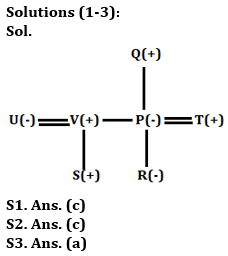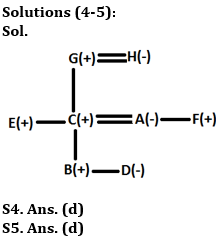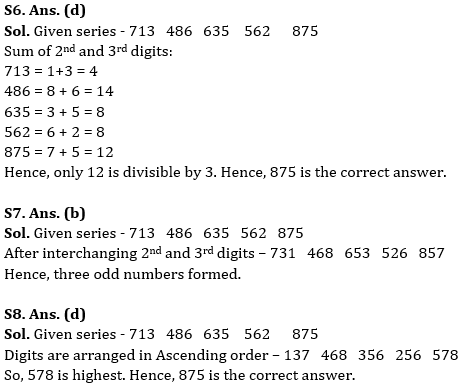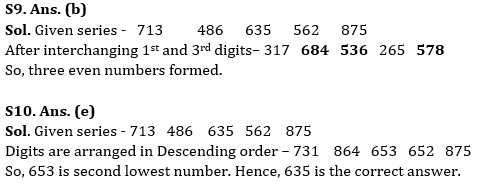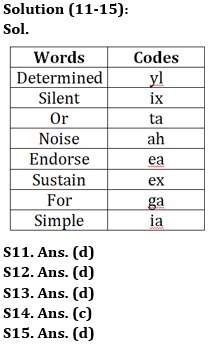## FAQs

### What is the selection process of the Bank Clerk?

The selection process of the Bank Clerk is Prelims & Mains.

#### Congratulations!Union Budget 2023-24: Free PDF